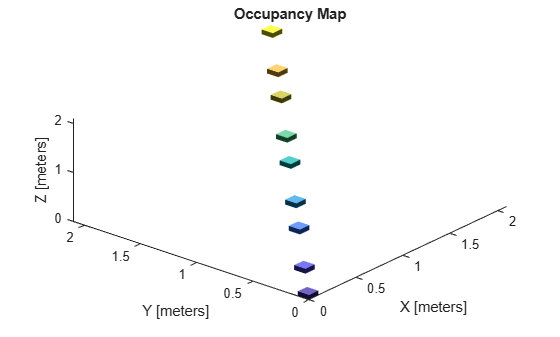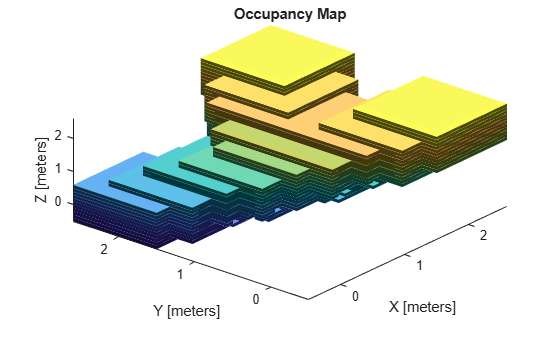# insertPointCloud

Insert 3-D points or point cloud observation into map

Since R2019b

## Syntax

``insertPointCloud(map3D,pose,points,maxrange)``
``insertPointCloud(map3D,pose,ptcloud,maxrange)``

## Description

example

````insertPointCloud(map3D,pose,points,maxrange)` inserts one or more sensor observations at the given `points` in the occupancy map, `map3D`. Occupied points are updated with an observation of 0.7. All other points between the sensor `pose` and `points` are treated as obstacle-free and updated with an observation of 0.4. Points outside `maxrange` are not updated. `NaN` values are ignored.```
````insertPointCloud(map3D,pose,ptcloud,maxrange)` inserts a `ptcloud` object into the map.```

## Examples

collapse all

The `occupancyMap3D` object stores obstacles in 3-D space, using sensor observations to map an environment. Create a map and add points from a point cloud to identify obstacles. Then inflate the obstacles in the map to ensure safe operating space around obstacles.

Create an `occupancyMap3D` object with a map resolution of 10 cells/meter.

`map3D = occupancyMap3D(10);`

Define a set of 3-D points as an observation from a pose `[x y z qw qx qy qz]`. This pose is for the sensor that observes these points and is centered on the origin. Define two sets of points to insert multiple observations.

```pose = [ 0 0 0 1 0 0 0]; points = repmat((0:0.25:2)', 1, 3); points2 = [(0:0.25:2)' (2:-0.25:0)' (0:0.25:2)']; maxRange = 5; ```

Insert the first set of points using `insertPointCloud`. The function uses the sensor pose and the given points to insert observations into the map. The colors displayed correlate to the height of the point only for illustrative purposes.

```insertPointCloud(map3D,pose,points,maxRange) show(map3D)```Insert the second set of points. The ray between the sensor pose (origin) and these points overlap points from the previous insertion. Therefore, the free space between the sensor and the new points are updated and marked as free space.

```insertPointCloud(map3D,pose,points2,maxRange) show(map3D)```Inflate the map to add a buffer zone for safe operation around obstacles. Define the vehicle radius and safety distance and use the sum of these values to define the inflation radius for the map.

```vehicleRadius = 0.2; safetyRadius = 0.3; inflationRadius = vehicleRadius + safetyRadius; inflate(map3D, inflationRadius); show(map3D)```## Input Arguments

collapse all

3-D occupancy map, specified as a `occupancyMap3D` object.

Points of point cloud in sensor coordinates, specified as an n-by-3 matrix of `[x y z]` points, where n is the number of points in the point cloud.

Point cloud reading, specified as a `pointCloud` object.

Note

Using `pointCloud` objects requires Computer Vision Toolbox™.

Position and orientation of vehicle, specified as an ```[x y z qw qx qy qz]``` vector. The vehicle pose is an xyz-position vector with a quaternion orientation vector specified as `[qw qx qy qz]`.

Maximum range of point cloud sensor, specified as a scalar. Points outside this range are ignored.

## Version History

Introduced in R2019b